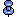Mathematical functions

Mathematical functions return values commonly needed for operations on mathematical data. Mathematical function names are not keywords.

Each function also accepts arguments that can be implicitly converted to the specified type. For example, functions that accept approximate numeric types also accept integer types. Adaptive Server automatically converts the argument to the desired type.

The mathematical functions are:

• abs

• acos

• asin

• atan

• atn2

• ceiling

• cos

• cot

• degrees

• exp

• floor

• log

• log10

• pi

• power

• rand

• round

• sign

• sin

• sqrt

• tan

Error traps are provided to handle domain or range errors of these functions. Users can set the arithabort and arithignore options to determine how domain errors are handled:

• arithabort arith_overflow specifies behavior following a divide-by-zero error or a loss of precision. The default setting, arithabort arith_overflow on, rolls back the entire transaction or aborts the batch in which the error occurs. If you set arithabort arith_overflow off, Adaptive Server aborts the statement that causes the error, but continues to process other statements in the transaction or batch.

• arithabort numeric_truncation specifies behavior following a loss of scale by an exact numeric type during an implicit datatype conversion. (When an explicit conversion results in a loss of scale, the results are truncated without warning.) The default setting, arithabort numeric_truncation on, aborts the statement that causes the error, but continues to process other statements in the transaction or batch. If you set arithabort numeric_truncation off, Adaptive Server truncates the query results and continues processing.

• By default, the arithignore arith_overflow option is turned off, causing Adaptive Server to display a warning message after any query that results in numeric overflow. Set the arithignore option on to ignore overflow errors.The arithabort and arithignore options have been redefined for release 10.0 or later. If you use these options in your applications, examine them to be sure they still produce the desired effects.